## LetsPlayMaths.Com

WELCOME TO THE WORLD OF MATHEMATICS

# Class-8 Parallelogram

Introduction to Parallelogram

Properties of Parallelogram

Parallelogram MCQ

Parallelogram Worksheets

## Introduction to Parallelogram

A quadrilateral having both pairs of opposite sides are parallel is known as parallelogram.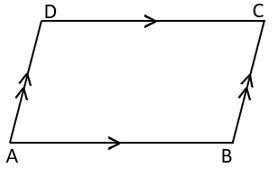In the above figure, ABCD is a quadrilateral in which AB || DC and AD || BC. Hence ABCD is a parallelogram.

## Properties of Parallelogram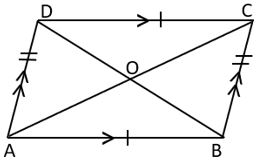1. Opposite sides are parallel i.e., AB || DC and AD || BC
2. Opposite sides are equal i.e., AB = CD and AD = BC
3. Opposite angles are equal i.e., ∠A = ∠C and ∠B = ∠D
4. Adjacent angles are supplementary i.e., ∠A + ∠B = 180°, ∠B + ∠C = 180°, ∠C + ∠D = 180°, ∠D + ∠A = 180°
5. Each diagonal bisects the parallelogram into congruent triangles.

Example 1. In a parallelogram ABCD, if ∠A = 80°, then find ∠B, ∠C, and ∠D.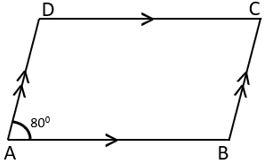Solution. As we know adjacent angles are supplementary of a parallelogram

∠A + ∠B = 180°

=> 80° + ∠B = 180°

=> ∠B = 180° − 80°

=> ∠B = 100°

Since the opposite angles of a parallelogram is equal, we have

∠A = ∠C = 80° and ∠B = ∠D = 100°

Hence, ∠B = 100°, ∠C = 80° and ∠D = 100°.

Example 2. Two adjacent angles of a parallelogram are as 4 : 5. Find the measure of each of its angles.

Solution. Let ABCD be the given parallelogram.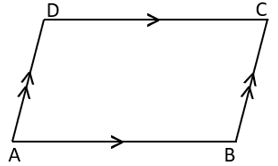Then ∠ and ∠ are its adjacent angles.

Let's assume ∠A = (4a)° and ∠B = (5a)°

As we know adjacent angles are supplementary of a parallelogram.

∠A + ∠B = 180°

=> 4a + 5a = 180°

=> 9a = 180°

=> a = 180°9

=> a = 20°

∠A = 4 × 20° = 80°

∠B = 5 × 20° = 100°

Since the opposite angles of a parallelogram is equal, we have

∠A = ∠C = 80°

∠B = ∠D = 100°

Hence, ∠A = 80°, ∠B = 100°, ∠C = 80°, and ∠D = 100°

Example 3. In the below given figure, ABCD is a parallelogram in which ∠BAD = 65° and ∠CBD = 85°. Calculate ∠ADB and ∠CDB.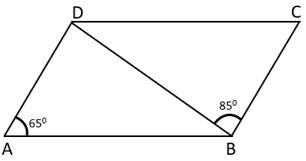Solution. As we know opposite angles of a parallelogram is equal.

Adjacent angles of a parallelogram are supplementary.

=> ∠BAD + ∠ABD + ∠CBD = 180°

=> 65° + ∠ABD + 85° = 180°

=> ∠ABD + 150° = 180°

=> ∠ABD = 180° − 150°

=> ∠ABD = 30°

Addition of all the angles of a triangle is 180°.

=> 65° + 30° + ∠ADB = 180°

=> ∠ADB + 95° = 180°

=> ∠ADB = 180° − 95°

∠ADC = ∠ABC = 85° + 30°

=> ∠ADB + ∠CDB = 115°

=> 85° + ∠CDB = 115°

=> ∠CDB = 115° − 85°

=> ∠CDB = 30°

Hence, ∠ADB = 85° and ∠CDB = 30°

Example 4. In the below given figure, ABCD is a parallelogram in which ∠CAD = 45°, ∠BAC = 30° and ∠COD = 120°. Find the value of ∠ABD, ∠BDC, ∠ACB and ∠CBD.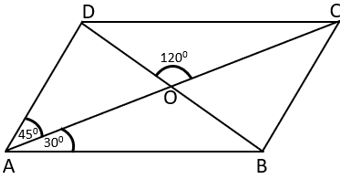Solution. As we know vertical opposite angles are equal.

∠COD = ∠AOB = 120°

Sum of all angles of a triangle is equal to 180°.

∠AOB + ∠BAO + ∠ABO = 180°

=> 120° + 30° + ∠ABO = 180°

=> 150° + ∠ABO = 180°

=> ∠ABO = 180° − 150°

=> ∠ABO = 30°

∠ABO = ∠ABD = 30°

AB || DC and BD is a transversal.

Hence, ∠BDC = ∠ABD = 30°

AD || BC and AC is a transversal.

Hence, ∠ACB = CAD = 45°

Opposite angles of a parallelogram are equal.

Hence, ∠BCD = ∠BAD = 45° + 30° = 75°

Sum of all angles of a triangle is equal to 180°.

In triangle BCD, ∠CBD + ∠BDC + ∠BCD = 180°.

=> ∠CBD + 30° + 75° = 180°

=> ∠CBD + 105° = 180°

=> ∠CBD = 180° − 105°

=> ∠CBD = 75°

Hence, ∠ABD = 30°, ∠BDC = 30°, ∠ACB = 45° and ∠CBD = 75°.

## Class-8 Parallelogram MCQ

Parallelogram Test - 1

Parallelogram Test - 2

## Class-8 Parallelogram Worksheets

Parallelogram Worksheet - 1

Parallelogram Worksheet - 2